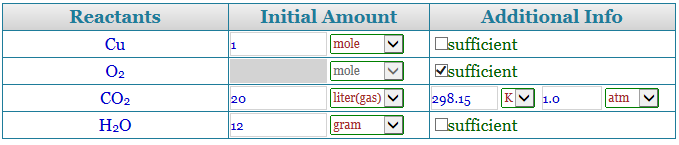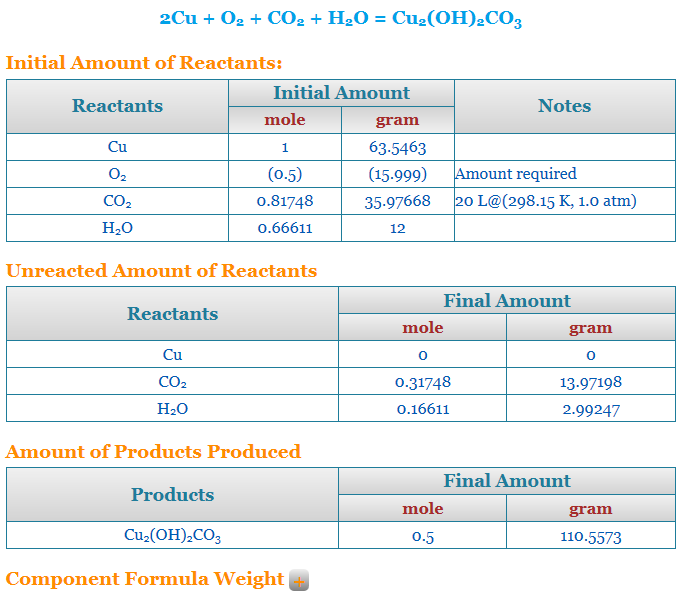Input a Reaction Equation

Calculation Type:

How to use the calculatorShow Me

1) Input a reaction equation to the box. No balance necessary. Example:
Cu + O2 + CO2 + H2O = Cu2(OH)2CO3

2) Select a Calculation Type. An input table will be created.

If you have information about one or more reactants, select Reactant Amount Given; Otherwise, select Product Amount Given.

3) Input amount available. Check 'sufficient' box if amount of a reactant is unknown.4) Click the 'Calculate' button. Calculation results will appear as below.Sample Equations

LiCoO2 = Li0.25CoO2 + Li{+} + e{-}
CuSO4*3.5H2O = CuSO4 + H2O
K4[Fe(CN)6] + CuSO4 = Cu2[Fe(CN)6] + K2SO4
Al2Si2O5(OH)4 + SiO2 + Mg{+2} = H2O +H{+} + Al7/3Si11/3O10(OH)2Mg1/6

Tip: use formula (O2)0.21*(N2)0.79 for air